# Classifying triangles

### Classifying triangles

#### Lessons

• Introduction
a)
Naming types of triangles based on their properties
1. By angles
• Acute
• Right
• Obtuse
2. By sides
• Scalene
• Isosceles
• Equilateral

• 1.
Classify the triangle by its sides and angles.
a)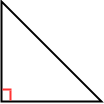b)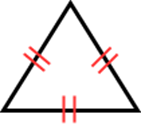c)d)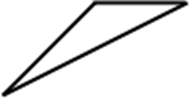• 2.
Draw and classify the triangle by its vertices. Then, determine if it is a right triangle.
a)
A(3, 6), B(3, 1), C(9, 1)

b)
A(2, 4), B(5, 10), C(5, -2)

c)
A(8, 7), B(11, 3), C(4, 2)

• 3.
Find x. Then, classify the triangle by its angles.
a)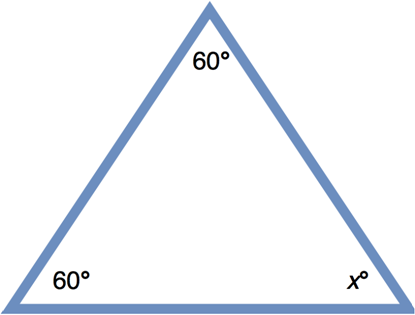b)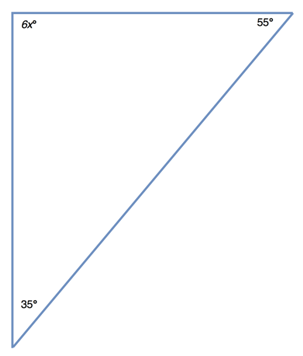c)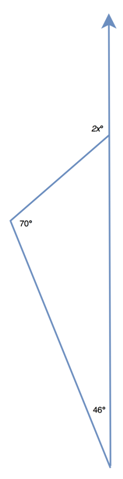• 4.
One angle of a triangular chocolate box is 20°. The measure of the second angle is 3 times smaller than the third one. Find the measures of the two unknown angles.